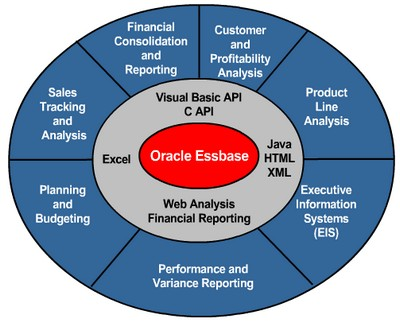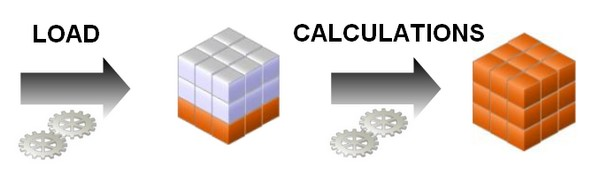# Essbase - Time balance properties

The tags :

• first,
• last,
• average,
• and expense

are available exclusively for use with accounts dimension members.

Using this tag requires an accounts dimension and a time dimension

If an accounts dimension member uses the time balance property, it affects how Essbase calculates the parent of that member in the time dimension. By default, a parent in the time dimension is calculated based on the consolidation and formulas of its children. For example, in the Sample.Basic database, the Qtr1 member is the sum of its children (Jan, Feb, and Mar). However, setting a time balance property causes parents, for example Qtr1, to roll up differently.

If you set the time balance as first, last, or average, set the skip property to tell Essbase what to do when it encounters missing values or values of 0.

## Accounts Member Tags

Tags Description
Time Balance Last The value for the last child member is carried to the parent. For example, March is carried to Qtr1.
Time Balance First The value for the first child is carried to the parent. For example, Jan is carried to Qtr1.
Time Balance Average The value of the average of the children is carried to the parent.

## Example

Dimensions Jan Feb Mar Qtr1 Year
Accounts Member1 11 12 13 36 Qtr1 + Qtr2 + Qtr3 + Qtr4
Accounts Member2 (TB First) 20 25 21 20 20
Accounts Member3 (TB Last) 25 21 30 30 Value of Qtr4

Normally, the calculation of a parent in the time dimension is based on the consolidation and formulas of children of the parent. However, if a member in an accounts branch is marked as TB First, any parent in the time dimension matches the member marked as TB First.

### Example of Time Balance as None

”None” is the default value. When you set the time balance property as none, Essbase rolls up parents in the time dimension in the usual way—the value of the parent is based on the formulas and consolidation properties of its children.

### Example of Time Balance as First

Set the time balance as “first” when you want the parent value to represent the value of the first member in the branch (often at the beginning of a time period).

For example, assume that a member named OpeningInventory represents the inventory at the beginning of the time period. If the time period was Qtr1, OpeningInventory represents the inventory at the beginning of Jan; that is, the OpeningInventory for Qtr1 is the same as the OpeningInventory for Jan. For example, if you had 50 cases of Cola at the beginning of Jan, you also had 50 cases of Cola at the beginning of Qtr1.

Tag OpeningInventory as first, as shown in the following example consolidation:

``````OpeningInventory (TB First), Cola, East, Actual, Jan(+),  50
OpeningInventory (TB First), Cola, East, Actual, Feb(+),  60
OpeningInventory (TB First), Cola, East, Actual, Mar(+),  70
OpeningInventory (TB First), Cola, East, Actual, Qtr1(+), 50
```
```

### Example of Time Balance as Last

Set the time balance as “last” when you want the parent value to represent the value of the last member in the branch (often at the end of a time period).

For example, assume that a member named EndingInventory represents the inventory at the end of the time period. If the time period is Qtr1, EndingInventory represents the inventory at the end of Mar; that is, the EndingInventory for Qtr1 is the same as the EndingInventory for Mar. For example, if you had 70 cases of Cola at the end of Mar, you also had 70 cases of Cola at the end of Qtr1.

Tag EndingInventory as last, as shown in the following example consolidation:

``````EndingInventory (TB Last), Cola, East, Actual, Jan(+),  50
EndingInventory (TB Last), Cola, East, Actual, Feb(+),  60
EndingInventory (TB Last), Cola, East, Actual, Mar(+),  70
EndingInventory (TB Last), Cola, East, Actual, Qtr1(+), 70
```
```

### Example of Time Balance as Average

Set the time balance as “average” when you want the parent value to represent the average value of its children.

For example, assume that a member named AverageInventory represents the average of the inventory for the time period. If the time period was Qtr1, then AverageInventory represents the average of the inventory during Jan, Feb, and Mar.

Tag AverageInventory as average, as shown in the following example consolidation:

``````AverageInventory (TB Average), Cola, East, Actual, Jan(+),  60
AverageInventory (TB Average), Cola, East, Actual, Feb(+),  62
AverageInventory (TB Average), Cola, East, Actual, Mar(+),  67
AverageInventory (TB Average), Cola, East, Actual, Qtr1(+), 63
```
```

## Skip

If you set the time balance as first, last, or average, set the skip property to tell Essbase what to do when it encounters missing values or values of 0.

### Tag / Properties / Setting

Setting Essbase Action
None Does not skip data when calculating the parent value.
Missing Skips #MISSING data when calculating the parent value.
Zeros Skips data that equals zero when calculating the parent value.
Missing and Zeros Skips #MISSING data and data that equals zero when calculating the parent value.

### Example

If you mark a member as last with a skip property of missing or missing and zeros, the parent of that time period matches the last nonmissing child. In the following example, EndingInventory is based on the value for Feb, because Mar does not have a value.

``````Cola, East, Actual, Jan, EndingInventory (Last),  60
Cola, East, Actual, Feb, EndingInventory (Last),  70
Cola, East, Actual, Mar, EndingInventory (Last),  #MI
Cola, East, Actual, Qtr1, EndingInventory (Last), 70
```
```

## Documentation / Reference

Discover MoreEssbase - (Accounts|Measures) Dimensions

Accounts dimensions are dimension that contains items that you want tomeasure, and makes Essbase built-in accounting functionality available. Accounting classifications. Ex: income expense profit...Essbase - Calculations

Essbase includes powerful calculation features for demanding analytic requirements. A rich library of functions makes it easy to define advanced and sophisticated business logic and relationships. Essbase...Essbase - The OLAP Design Cycle (to create an optimized database)

A contains two types of values : the values that you enter or load (input data) and the values that are calculated from the input data (calculated data) Then a typical conforms to the following...Essbase - Time dimensions (Year)

Tag a dimension as time if it contains members that describe how often you collect and update data. In the Sample.Basic database, for example, the Year dimension is tagged as time, as are its descendants—all...